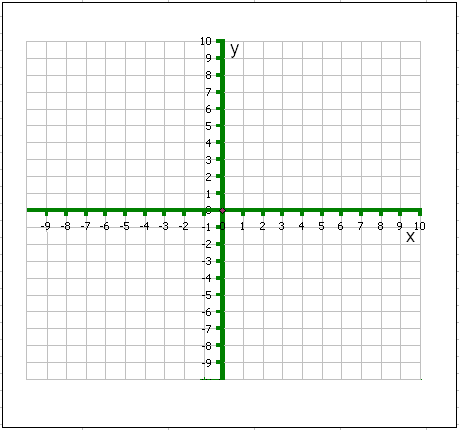### Graphing exercise II: Name: ___________

Making graphs from functions using a spreadsheet

1. Graphing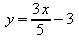for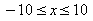: Open up spreadsheet software such as Microsoft Excel or OpenOffice Calc in the laboratory.
2. In cell A1 enter x
3. In cell B1 enter y
4. In cell A2 put -10
5. In cell A3 put -9
6.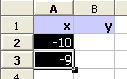Select the cells A2 to A3 by dragging across them:
7. Click on the small black square in the lower right hand corner (this is called the fill control).
8. While holding the mouse button down, drag down to A22.
9. In B2 type: =(3* A2 /5)-3
10. Fill this formula down to B22 using the fill control.
11. Select both columns from A1 to B22.
12.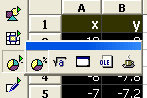Click on the chart wizard in Excel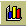or Calc
13. Use the wizard and demonstrated in class to set up an x-y scatter chart of the data.
14. Copy the graph onto the blank graph further below.
15. Does it match the graph you made Tuesday?
16. Use a new blank sheet and graph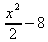for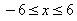.
17.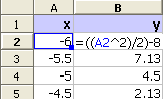In cell A1 enter x
18. In cell B1 enter y
19. In cell A2 put -6
20. In cell A3 put -5.5
21. Drag down across the center of A2 and A3 and then use the fill control to fill down to A26.
22. Note that the exponentiation symbol ^ is obtained from "shift-6".
23. In B2 type: =((A2^2)/2)-8
24. Fill this formula down to B26 using the fill control.
25. Make an x-y scatter chart of the data using the chart wizard.
26. Is the line a "smooth curve?"
27. Copy the graph onto the blank graph further below.
28. Does it match the graph you made Tuesday?
29. Use a new blank sheet and graph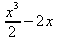for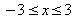with an interval of 0.5 (fill down to A14) using the spreadsheet function =((A2^3)/2)-2*A2
30. Is the line a "smooth curve?"
31. Copy the graph onto the blank graph further below.
32. Does it match the graph you made Tuesday?